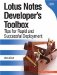# How to Zero Pad a Text Number

This routine illustrates how to zero pad a text number. This solution might be used when creating an alphanumeric document record number (refer to the section entitled "Create a Unique Document Record Number" for additional information).

How It Works

This solution compares the string length of value (num) with a total number of digits required (requiredDigits). If the difference is less than the required value, zeros are prefixed to the value by using the String function and concatenating it with the original value. To adjust the number of zeros padded to the number, increase or decrease the numeric value assigned to requiredDigits.

Implementation

To implement this solution, set the total number of digits required (see Figure 13.22) and assign a value to the num object. In the example, the prompt statement is used to assign a value to the num object.

```Dim w As New NotesUIWorkspace
Dim num As String
Dim diff As Integer
Dim requiredDigits As Integer

'---------------------------------------
' Specify total number of digits required.
' A number less than this value will be
'---------------------------------------
requiredDigits = 4

'---------------------------------------
' Prompt user to specify a value
'---------------------------------------
num = Trim(w.Prompt (3, "Enter a value",+_
"Specify a number to zero pad?"))

'---------------------------------------
' Compare string lengths and pad with zeros
'---------------------------------------
diff = requiredDigits - Len(num)
If Len (num) < requiredDigits Then
num = String (diff, "0") + num
End If
Msgbox "This is a zero padded number: " + num,, "Padded Value"
```

Figure 13.22. Pad a text number with zerosLotus Notes Developers Toolbox: Tips for Rapid and Successful Deployment
ISBN: 0132214482
EAN: 2147483647
Year: N/A
Pages: 293
Authors: Mark Elliott